# Calculus 1 : How to find acceleration

## Example Questions

1 2 27 28 29 30 31 32 33 35 Next →

### Example Question #341 : How To Find Acceleration

The position of a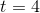is given by the following functions: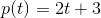Find the acceleration.Explanation:

In order to find the acceleration of a certain point, you first find the derivative of the position function to get the velocity function and the derivative of the velocity function to get the acceleration function: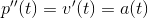In this case, the position function is:Then take the derivative of the position function to get the velocity function: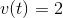Then take the derivative of the velocity function to get the acceleration function: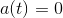Then, pluginto the acceleration function: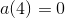Therefore, the answer is:### Example Question #342 : How To Find Acceleration

Given the position function of an object in motion (in meters), find the acceleration of the object at t=2 seconds.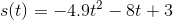-4.9 m/s/s

9.8 m/s/s

-9.8 m/s/s

4.9 m/s/s

-9.8 m/s/s

Explanation:

The acceleration of an object in motion at any given time is modeled by the second derivative of its position function. That is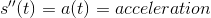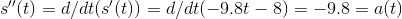The acceleration of the object at t=2 seconds is then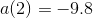In this case, the acceleration is constant across all values of t.

### Example Question #343 : How To Find Acceleration

Find the acceleration of a particle at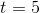given the following velocity function: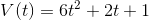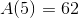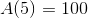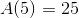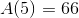Explanation:

The first step is to obtain the acceleration equation.

This is done by taking the first derivative of the velocity function,using the power rule,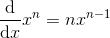.

The acceleration equation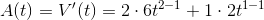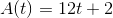can be used to find the acceleration at any given time.

In our case, plugging in 5 for t into the equation gives,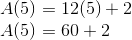.

### Example Question #344 : How To Find Acceleration

Find the acceleration of a particle given its velocity function is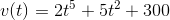.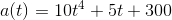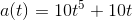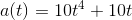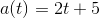Explanation:

The acceleration function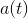can be found given any velocity function by taking its derivative.

Using the power rulethe derivative becomes,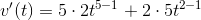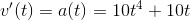.

Recall that the derivative of a constant is always zero.

### Example Question #341 : How To Find Acceleration

Find the acceleration function of a car who's velocity function is given by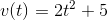.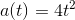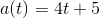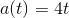Explanation:

The derivative of the velocity function is acceleration, and using the power rule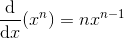,

you get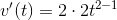.

Recall that the derivative of a constant is zero.

1 2 27 28 29 30 31 32 33 35 Next →